• 总结matplotlib绘图如何设置坐标轴刻度大小和刻度。上代码：from pylab import *from matplotlib.ticker import MultipleLocator, FormatStrFormatterxmajorLocator = MultipleLocator(20) #将x主刻度标签设置为20的...
总结matplotlib绘图如何设置坐标轴刻度大小和刻度。
上代码：
from pylab import *
from matplotlib.ticker import MultipleLocator, FormatStrFormatter
xmajorLocator = MultipleLocator(20) #将x主刻度标签设置为20的倍数
xmajorFormatter = FormatStrFormatter('%1.1f') #设置x轴标签文本的格式
xminorLocator = MultipleLocator(5) #将x轴次刻度标签设置为5的倍数
ymajorLocator = MultipleLocator(0.5) #将y轴主刻度标签设置为0.5的倍数
ymajorFormatter = FormatStrFormatter('%1.1f') #设置y轴标签文本的格式
yminorLocator = MultipleLocator(0.1) #将此y轴次刻度标签设置为0.1的倍数
t = arange(0.0, 100.0, 1)
s = sin(0.1*pi*t)*exp(-t*0.01)
ax = subplot(111) #注意:一般都在ax中设置,不再plot中设置
plot(t,s,'--b*')
#设置主刻度标签的位置,标签文本的格式
ax.xaxis.set_major_locator(xmajorLocator)
ax.xaxis.set_major_formatter(xmajorFormatter)
ax.yaxis.set_major_locator(ymajorLocator)
ax.yaxis.set_major_formatter(ymajorFormatter)
#显示次刻度标签的位置,没有标签文本
ax.xaxis.set_minor_locator(xminorLocator)
ax.yaxis.set_minor_locator(yminorLocator)
ax.xaxis.grid(True, which='major') #x坐标轴的网格使用主刻度
ax.yaxis.grid(True, which='minor') #y坐标轴的网格使用次刻度
show()
绘图如下：如果仔细看代码，可以得知，设置坐标轴刻度和文本主要使用了"MultipleLocator"、"FormatStrFormatter"方法。
这两个方法来自matplotlib安装库里面ticker.py文件；"MultipleLocator(Locator)"表示将刻度标签设置为Locator的倍数,"FormatStrFormatter"表示设置标签文本的格式，代码中"%1.1f"表示保留小数点后一位，浮点数显示。
相应的方法还有：除了以上方法，还有另外一种方法，那就是使用xticks方法(yticks，x,y表示对应坐标轴)，xticks用法可在python cmd下输入以下代码查看:
import matplotlib.pyplot as plt
help(plt.xticks)
代码如下：
import numpy as np
import matplotlib.pyplot as plt
fig,ax = plt.subplots()
x = [1,2,3,4,5]
y = [0,2,5,9,15]
#ax is the axes instance
group_labels = ['a', 'b','c','d','e']
plt.plot(x,y)
plt.xticks(x, group_labels, rotation=0)
plt.grid()
plt.show()
绘图如下：上图中使用了"plt.xticks"方法设置x轴文本，标签文本使用group_labels中的内容，因此可以根据需要修改group_labels中的内容。
网上看到的另一种方法，代码如下:
import matplotlib.pyplot as pl
import numpy as np
from matplotlib.ticker import MultipleLocator, FuncFormatter
x = np.arange(0, 4*np.pi, 0.01)
y = np.sin(x)
pl.figure(figsize=(10,6))
pl.plot(x, y,label="$sin(x)$")
ax = pl.gca()
def pi_formatter(x, pos):
"""
比较罗嗦地将数值转换为以pi/4为单位的刻度文本
"""
m = np.round(x / (np.pi/4))
n = 4
if m%2==0: m, n = m/2, n/2
if m%2==0: m, n = m/2, n/2
if m == 0:
return "0"
if m == 1 and n == 1:
return "$\pi$"
if n == 1:
return r"$%d \pi$" % m
if m == 1:
return r"$\frac{\pi}{%d}$" % n
return r"$\frac{%d \pi}{%d}$" % (m,n)
# 设置两个坐标轴的范围
pl.ylim(-1.5,1.5)
pl.xlim(0, np.max(x))
# 设置图的底边距
pl.grid() #开启网格
# 主刻度为pi/4
ax.xaxis.set_major_locator( MultipleLocator(np.pi/4) )
# 主刻度文本用pi_formatter函数计算
ax.xaxis.set_major_formatter( FuncFormatter( pi_formatter ) )
# 副刻度为pi/20
ax.xaxis.set_minor_locator( MultipleLocator(np.pi/20) )
# 设置刻度文本的大小
for tick in ax.xaxis.get_major_ticks():
tick.label1.set_fontsize(16)
pl.legend()
pl.show()
绘图如下：以上就是本次小编整理的全部内容，感谢你对脚本之家的支持。
展开全文• 在开始本文之前，不熟悉的朋友可以先看看这篇文章：Python Matplotlib库入门指南 本文主要介绍的是关于Python利用matplotlib.pyplot绘图设置坐标轴刻度的相关内容，分享出来供大家参考学习，下面话不多说了，来一起...
前言
matplotlib.pyplot是一些命令行风格函数的集合，使matplotlib以类似于MATLAB的方式工作。每个pyplot函数对一幅图片(figure)做一些改动：比如创建新图片，在图片创建一个新的作图区域(plotting area)，在一个作图区域内画直线，给图添加标签(label)等。matplotlib.pyplot是有状态的，亦即它会保存当前图片和作图区域的状态，新的作图函数会作用在当前图片的状态基础之上。
在开始本文之前，不熟悉的朋友可以先看看这篇文章：Python Matplotlib库入门指南
本文主要介绍的是关于Python利用matplotlib.pyplot绘图设置坐标轴刻度的相关内容，分享出来供大家参考学习，下面话不多说了，来一起看看详细的介绍吧。
问题描述：
在使用matplotlib绘图时，可能会需要设置坐标轴上刻度之间的距离，或者为刻度设置标签。
技术要点：
pyplot的xticks()和yticks()函数的用法。
参考代码1：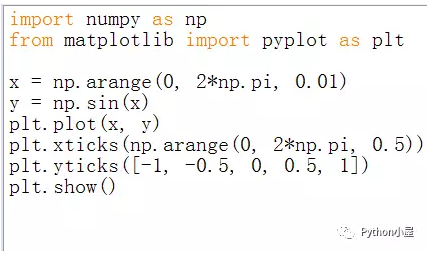运行结果1：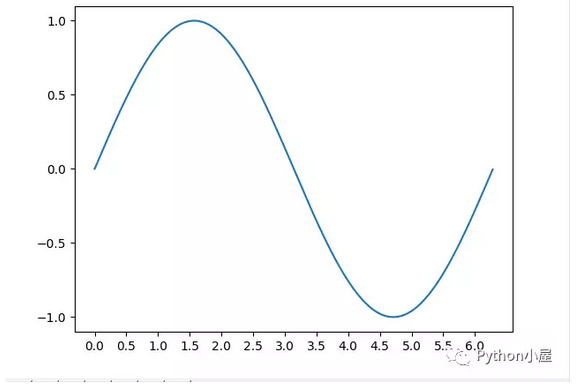参考代码2：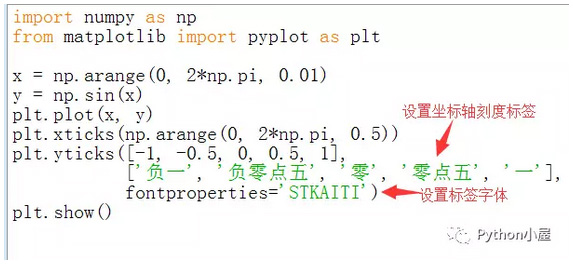运行结果2：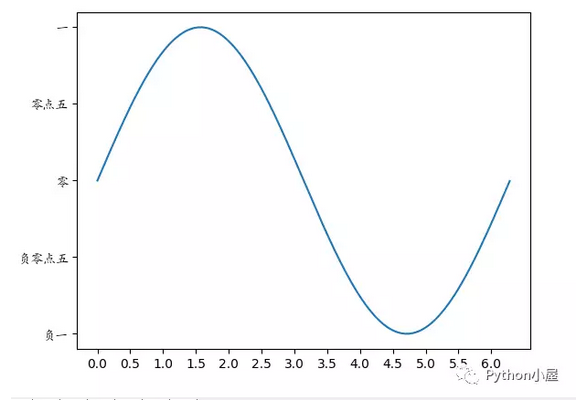最后给学习python的新手们推荐一个视频：Let's python从入门到精通系列视频教程  ，该视频讲解的非常清楚，如果你想走进Python行业，通过一些不错的视频开始学习是个不错建议。
总结
以上就是这篇文章的全部内容了，希望本文的内容对大家的学习或者工作具有一定的参考学习价值，如果有疑问大家可以留言交流，谢谢大家对本网站的支持。
展开全文• 首先，我们需要在 Python 中导入所需的库与模块，并对 notebook 环境与图表风格进行初步的设置： 运用 axes 函数，我们构建了一个空的坐标系，并将坐标轴刻度设置为幂形式： Matplotlib 中的 ticker 模块用于支持...
原标题：Python中坐标轴刻度的设置与调整丨数析学院
本节将向大家演示在 Python 中如何运用 Matplotlib 库设置图表中的坐标轴刻度。首先，我们需要在 Python 中导入所需的库与模块，并对 notebook 环境与图表风格进行初步的设置：运用 axes 函数，我们构建了一个空的坐标系，并将坐标轴刻度设置为幂形式：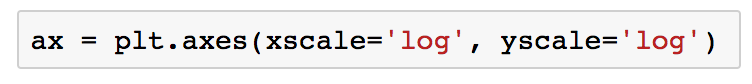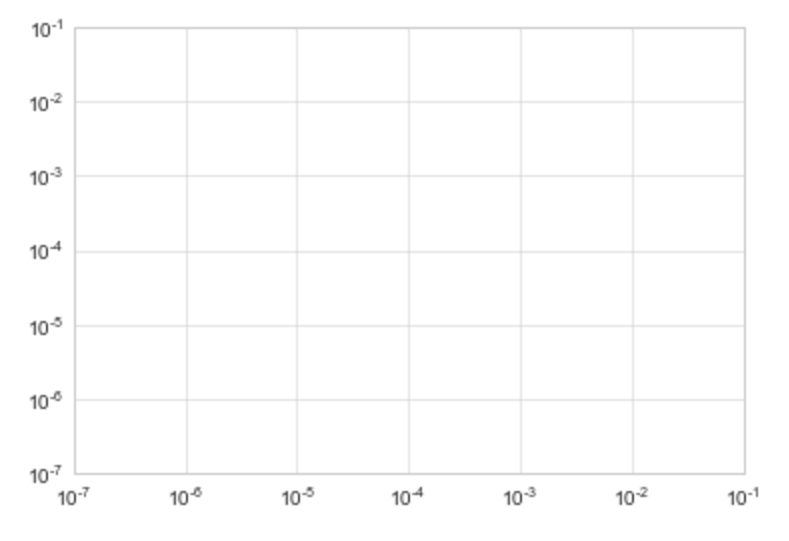Matplotlib 中的 ticker 模块用于支持坐标轴刻度的修改，调用下列命令可以初步查看主副坐标轴刻度的数值定位方式（locator）与具体格式（formatter）：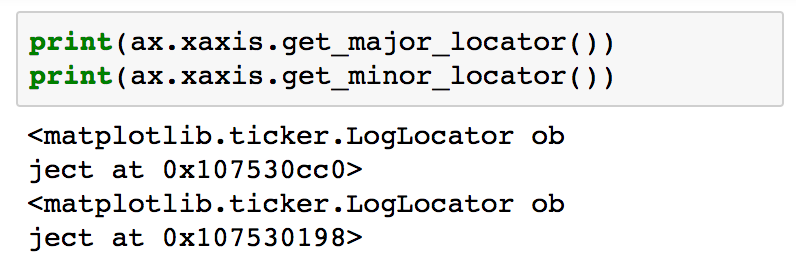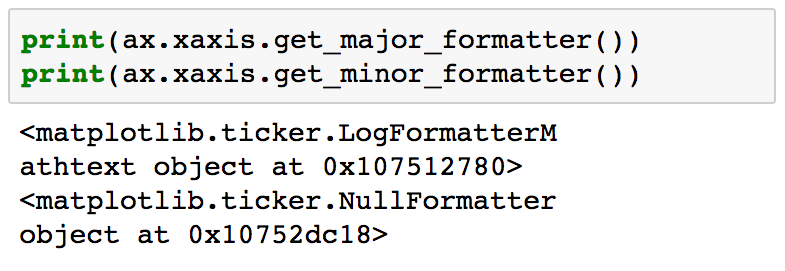我们可以使用 setmajorlocator 和 setmajorformatter 命令来调整坐标轴的刻度，如去除坐标轴的刻度显示：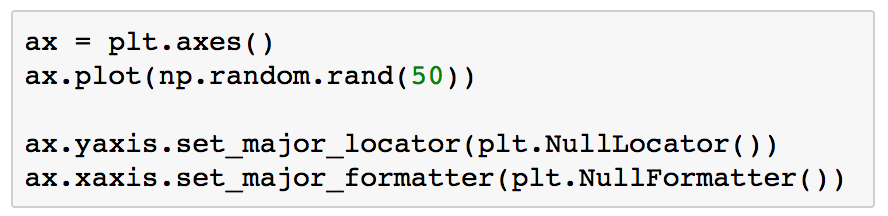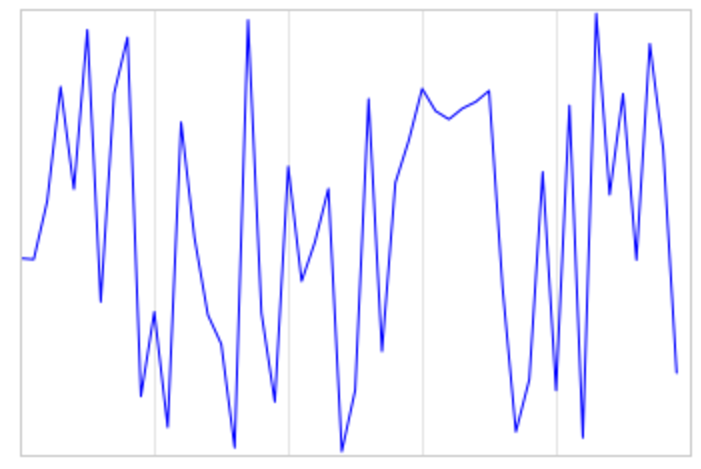责任编辑：
展开全文• Matplotlib是Python提供的一个二维绘图库，所有类型的平面图，包括直方图、散点图、折线图、点图、热图...本文主要介绍了关于Python利用matplotlib.pyplot绘图时如何设置坐标轴刻度的相关资料，需要的朋友可以参考下。
• 原博文2018-03-20 16:41 −学习python中matplotlib绘图设置坐标轴刻度、文本 http://www.jb51.net/article/134638.htm Python绘图 https://www.cnblogs.com/chaoren399/p/5792168.html ...相关推荐2019-12-24 21:15...
原博文
2018-03-20 16:41 −
学习python中matplotlib绘图设置坐标轴刻度、文本 http://www.jb51.net/article/134638.htm Python绘图 https://www.cnblogs.com/chaoren399/p/5792168.html ...
相关推荐
2019-12-24 21:15 −
1.算术运算
+ - * /
// —— 结果取整
% ——取余
** —— 幂
2.数学函数
round（）—— 四舍五入
abs（）&mdas...
2019-12-10 15:28 −
转自：https://blog.csdn.net/qq_36381299/article/details/80634451
前言：
根据搜索相关的职位，获取职位数量，由职位数量得到职位相关页码链接，再由相关页码链接获得每个职位链接，最后由职位链接获取详细的职位描述。以上获得链接和职位描述由正则表达式完...
2019-12-06 15:37 −
通过Object.defineProperty重新定义get和set,来实现数据的双向获取。
参考：https://www.jb51.net/article/143456.htm...
2019-12-20 14:47 −
JSON 函数
encode
Python encode() 函数用于将 Python 对象编码成 JSON 字符串。
语法
实例
以下实例将数组编码为 JSON 格式数据：
以上代码执行结果为：
...
2019-12-21 15:15 −
一、os.system方法
这个方法是直接调用标准C的system() 函数，仅仅在一个子终端运行系统命令，而不能获取命令执行后的返回信息。
os.system(cmd)的返回值。如果执行成功，那么会返回0，表示命令执行成功。否则，则是执行错误。
使用os.system返回值是脚本的退出状态码，该方法...
2019-12-05 15:34 −
1、删除文件打开后的缓冲文件。 os.system("/sbin/fuser -k .nfs*"); os.system("rm -rf .nfs*"); os.system("rm -rf .*swp"); 2、正则表达式去除某些选择 rm_dat = re.compile(r'...061
2019-12-04 22:17 −
使用matplotlib绘制图像
import matplotlib.pyplot as plt
from matplotlib.pyplot import MultipleLocator
import numpy as np
import seaborn as sns #描绘曲线图,可以对通过np...
2019-12-19 11:39 −
python 3.7 pycharm
1.安装selenium
pip3 install selenium
2.下载与chrome匹配的chromdriver.exe,放到项目的解释器路径下，跟python.exe同一个路径
随笔最后附上匹配列表和下载链接
注：...
2019-12-16 17:05 −
一、项目简介
1.1 项目博客地址
https://www.cnblogs.com/lmc7318/p/12050055.html
1.2 项目完成的功能与特色
爬虫爬取天气后报网站的厦门的历史天气，把爬取到的厦门历史天气保存到csv文件，然后提取c...
2019-12-23 01:33 −
对于包于模块的定义：http://www.imooc.com/article/247114这里写的还是不错的。
这个参考链接也很不错，讲的很清楚了为什么相对路径不能直接用脚本的形式执行。
https://www.jianshu.com/p/04bac02ae3f0
以前用P...
展开全文• matplotlib，利用pyplot与面向对象的api两种方式设置坐标轴刻度的个数以及标签matplotlib
• 总结Matplotlib绘图如何设置坐标轴刻度大小和刻度。 上代码： from pylab import * from matplotlib.ticker import MultipleLocator, FormatStrFormatter xmajorLocator = MultipleLocator(20) #将x主刻度标签...python matplotlib 文本
• serif']=['SimHei'] # 用来正常显示中文标签plt.rcParams['axes.unicode_minus']=False # 用来正常显示负号import pandas as pdimport numpy as np新建隐藏坐标轴from...
• 本篇文章给大家详细介绍了python中matplotlib绘图设置坐标轴刻度、文本等基本知识点，对此有兴趣的朋友学习下吧。
• 刻度设置参考文档：xticks 命令yticks 命令以xticks为例：matplotlib.pyplot.xticks(args, *kwargs)获取或者设置当前刻度位置和文本的 x-limits:return locs, labels where locs is an array of tick locations ...
• 1.去除横纵坐标值 plt.xticks([]) # 去掉横坐标值 plt.yticks([]) # 去掉纵坐标值 2.设置横纵坐标名称 ax1.set_xlabel('Output class',fontsize=24) #...3.设置坐标轴刻度大小 plt.tick_params(labelsize=14) ...python
• 7.1.设置坐标轴刻度 7.2.设置刻度标签（Setting Tick Labels） 7.3.调整刻度标签 (Adjusting the ticklabels) 7.设置刻度 7.1.设置坐标轴刻度 到目前为止，在所有例子中Matplotlib都自动负责了确定轴上间距点的任务...
• xsticks和ysticks函数的作用都是获取或设置坐标轴刻度及标签。其中 xsticks函数作用是获取或设置x坐标轴刻度及标签。 ysticks函数作用是获取或设置y坐标轴刻度及标签。 两者参数相同，仅功能稍有不同。 ...matplotlib 标签 ticks
• 欢迎关注”生信修炼手册”!在matplotlib中，通过子模块ticker可以对坐标轴刻度的位置和样式进行设置。刻度线分为major和minor ticks, 通过以下4个函数可以对其位...列表 字符串 css 编程语言 数据可视化
• pandas的plot函数已经可以满足很多的基本作图需求，但是其有一个比较明显缺点是不能一次性的在同一个坐标轴中画不同类型的图，比如折线图和柱状图，而且也不能设置坐标轴刻度的格式。当然这两个需求在matplotlib中是...
• matplotlib制图，坐标轴刻度间隔，MultipleLocatorpython matplotlib
• #刻度设置（分别设置x轴和y轴） y_tick = np.linspace(0,20,5) plt.yticks(y_tick,fontsize=20,color='#000000') plt.xticks([]) #不显示x轴刻度值 #x轴刻度旋转 #ax.set_xticklabels(ax.get_xticklabels(),rotation...
• 本来是想让横轴显示1-20的整数，刻度之间间隔1，但是画出图来却显示有浮点数 plt.title('train loss') plt.plot(np.arange(1,len(losses)+1), losses) plt.plot(np.arange(1,len(eval_losses)+1), eval_losses) 经...python 可视化
•python 数据可视化
• 总结matplotlib绘图如何设置坐标轴刻度大小和刻度。上代码：from pylab import *from matplotlib.ticker import MultipleLocator, FormatStrFormatterxmajorLocator = MultipleLocator(20) #将x主刻度标签设置为20的...
• 一、用默认设置绘制折线图 import matplotlib.pyplot as plt x_values=list(range(11)) #x的数字是0到10这11个整数 y_values=[x**2 for x in x_values] #y的数字是x数字的平方 plt.plot(x_values,y_values,...Python matplotlib
• 设置坐标轴刻度 设置坐标轴的范围 我们通常使用axis()来设置x轴、y轴的刻度范围。axis()待传人的参数分别为xmin, xmax, ymin, ymax。 import matplotlib.pyplot as plt x = [i for i in range(4)] y = [5, 4...数据可视化 python 可视化
• 利用matplotlib设置坐标轴刻度和次刻度。 （1）只显示次刻度标签位置，没有标签文本 from matplotlib.ticker import MultipleLocator, FormatStrFormatter xmajorLocator = MultipleLocator(a) #将x主刻度标签设置...python
• import matplotlib.pyplot as plt plt.xticks([0, 100, 200, 300, 400, 500, 600, 700]) plt.tick_params(labelsize=13) #刻度字体大小13
• 可以调用 xlable() 和 ylabel() 函数分别设置 X 轴、Y 轴的名称，也可以通过 title() 函数设置整个数据图...例如，如下程序为数据图添加了名称、标题和坐标轴刻度值：import matplotlib.pyplot as plt x_data = ['2...
• 用matplotlib画二维图像时，默认情况下的横坐标和纵坐标显示的值有时达不到自己的需求，需要借助xticks()和yticks()分别对横坐标x-axis和纵坐标y-axis进行设置。import numpy as npimport matplotlib.pyplot as pltx...
• 一、当我们用Python matplot时作图时，...二、借助matplotlib.ticker.FuncFormatter()，将坐标轴格式化。例子：# encoding=utf-8import matplotlib.pyplot as pltfrom matplotlib.ticker import FuncFormatterplt.r......